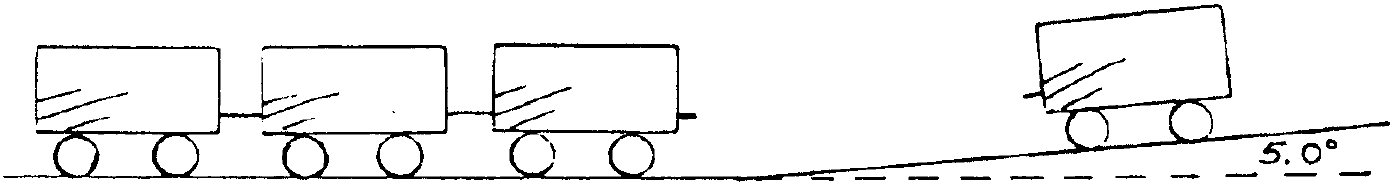2015-11-12

Problem Statement

In a train yard, train cars are rolled down a long hill in order to link them up with other cars as shown. A car of mass 4000. kg starts to roll from rest at the top of a hill 5.0 m high, and inclined at an angle of 5.0° to the horizontal. The coefficient of rolling friction between the train and the track is 0.050. What velocity would the car have if it linked up with 3 identical cars sitting on flat ground at the bottom of the track?

Hint: The equation for rolling friction is just like the one for sliding friction.

IllustrationMy Solution

The speed of the car after leaving the hill is equal to

v = sqrt(kinetic_energy * 2 / m)

And its kinetic energy will be equal to the work done on it:

kinetic_energy = F * d

Getting the distance is a matter of simple geometry:

d = csc (5°) * 5 m ~= 57.37 m

The force is a bit more complicated, note that I am using the net force, taking friction into account

F = tan (5°) * 4 * 10^4 N - 0.05 * sec (5°) * 4 * 10^4 N
= 4 * 10^4 N * (tan (5°) - 0.05 * sec (5°)) ~= 1,492 N

Throwing everything together

v = sqrt(F * d * 2 / m) ~= 6.542 m / s

As the velocity will be equally divided between the cars

v = (6.542 m / s) / 4 = 1.635 m / s

Thus, the answer is 1.64 m / s.

Note that I used 10 m s^-2 for the gravitational acceleration. If you want to be more precise, use 9.80665 m s^-2, the the standard acceleration due to gravity.